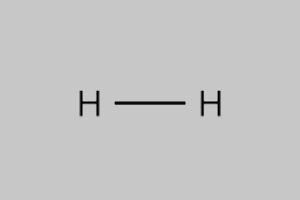# Hydrogen Gas Formula

Hydrogen gas formula is one of the simplest formulas found in chemistry. Hydrogen is basically the first element in the periodic table. It is described as a tasteless, colourless, odourless, nonmetallic, and highly flammable gas. It is also the lightest chemical element and found in abundance in stars. Around the 16th century, hydrogen gas was first artificially manufactured using the reaction between acids and metals. In the year 1766–81, an English scientist named Henry Cavendish was the first to determine that hydrogen gas was indeed a distinct or separate substance.

## Hydrogen Gas Chemical Formula

Hydrogen gas mainly consists of hydrogen atoms forming diatomic molecules. Therefore, the molecular formula of Hydrogen gas can be determined by observing the hydrogen atoms. Normally, a pair of hydrogen atoms are found bonded together into molecules. As a result, the formula is denoted as H2.

 Formula H2 Molar Mass 2.016 g/mol Density 0.0893 g/L Melting Point -259.2 °C Boiling Point -252.9 °C

### Hydrogen Gas Structural Formula

Below is the diagrammatic representation of hydrogen gas structural formula.Keep visiting BYJU’S to access pages of different formulas of important chemical compounds.

1. Beenish